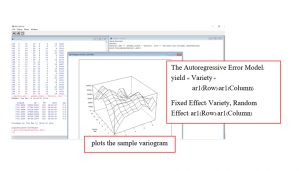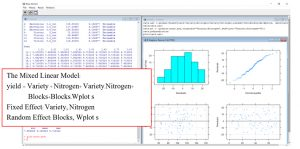# Restricted maximum likelihood

Maximum likelihood (REML) approach is a particular form of maximum likelihood  estimation which does not base estimates on a maximum likelihood fit of all the information, but instead uses a likelihood function calculated from a transformed set of data.

In the case of variance component estimation, the original data set is replaced by a set of contrast calculated from the data, and the likelihood function is calculated from the probability distribution of these contrasts, according to the model for the complete data set. In particular, REML is used as a method for fitting linear mixed model. In contrast to the earlier likelihood function estimation, REML can produce unbiased estimates of variance and covariance parameters

The REML algorithm provides several important types of analysis, that are useful in a wide range of application areas including biology, medicine, industry and finance. In biology they are usually known as linear mixed models, but in some application areas (e.g. education) they may be called multi-level models.

One of the key features of REML is that it can analyze data that involve more than one source of error variation. In this respects it is similar to the ANOVA algorithm, and the similarities and differences between the two methods are explored. An important advantage of REML over ANOVA is that it can analyze unbalanced designs. It also has a powerful prediction algorithm that extends the ideas in regression prediction algorithm to cover random as well as fixed effects.

REML for Meta analysis, showing a simultaneous analysis of several disparate data sets to obtain combined estimates for the treatments of interest. A further advantage of REML.

Model spatial correlations between observations in two-dimensions. The designs often contain too many varieties for the conventional blocking techniques) to be effective.

Analysis of repeated measurements, REML provides a powerful alternative to conventional methods such as repeated-measures ANOVA or the analysis of contrasts over time.### Measuring the size of single celled algae using flow fluorescence activated cell sort method (FACS)

ASreml provides restricted maximum likelihood mixed model to estimate the variance in size due clones compared to other environmental sources of variance.

Find the original publication

C. E. Seed, I. Larma and J. L. Tomkins, Algal Research, Volume 16, 2016, Pages 93–101.